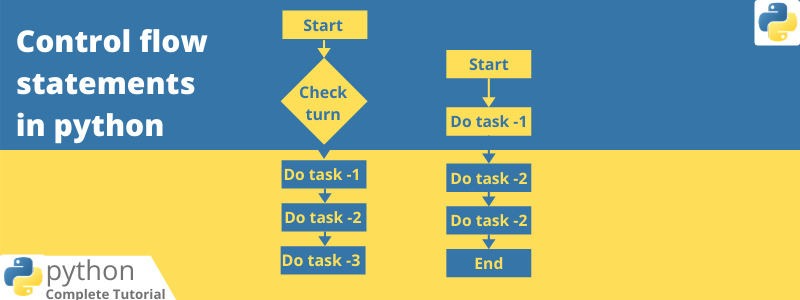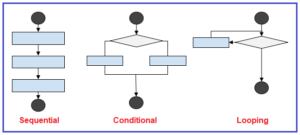# Flow Control Statement Of Python

## Topic – Flow Control Statements

### These are of 3 types :

1. Conditional Statements
2. Iterative Statements
3. Transfer Statements### Conditional Statements :

If we have to write a program in which we want to execute a code on behalf of conditions then we come for Conditional Statements.

We have 3 types of Conditional Statements :

1. if:
2. if-else:
3. if-elif-else:

### if :

In this statement we have only 1 condition. If the condition is true the program will be executed otherwise, it come out from the program.

Examples :

Q => How to write a program which executed if right name given ?

### Soln :

name = input(“Enter your Name :”)
if name ==‘keshav’:
print(“You print the right name”)

### if-else :

If condition is true 1st Action will be executed otherwise 2nd Action.

Example :

Q => Passed or fail percentage wise ?

### Soln :

if p>33 and p<100:
print(‘Pass’)
else:
print(‘Fail’)

### if-elif-else :

While using this statement we make a program with multiple condition.

Example :

Q =>Make a Voting age criteria program ?

### Soln :

if age<18:
print(‘You are under age\nYou are not eligible’)
elif age<=18 and age<90:
print(‘You are eligible for voting’)
else:
print(‘You are over age’)

## Iterative Statements :

These are the statements helps to execute a group of statements multiple times.

### There are 2 types of statements in python are :

1. for loop
2. while loop

Let’s  learn where these statements are to be used:

### for loop:

It is used to iterate over elements of a sequence or when you have a piece of code which you want to repeat “n” number of time.

Examples :

Q => If you want to print the no. from 1 to 100 ?

Soln :  Let, a is a variable in which we stored a datatype(Range) , with the help of            which we can simply print numbers.

a = range(1,100,1)
for i in a:
print(i)

Soln :

a = ‘Keshav’
for z in a:
print(z)

### Q => How to print the index value with list items ?

Soln : for this make a list of items after that read the code below:

a = [‘a’, ‘b’, ‘c’, ‘d’]
i = 0
for x in a:
print(i,x)
i = i+1

### Nested for loop :

for loop inside another for loop is known as Nested for loop.

Q => How to

Soln :

a = [‘Abhishek’,‘Nikhil’,‘Tarun’,‘Manyu’,‘Shivam’]
b = [‘How are You’]
for i in a:
print(i)
for j in b:
print(j)

## while loop:

The while loop in Python is used to iterate over a block of code as long as the test expression (condition) is true.

Examples :

Q => How to print a no. from 11 to 20 by using while loop ?

Soln :

x = 11
while x <=20:
print(x)
x = x+1

Q => How to display the sum of first n numbers ?

Soln :

n = 18
sum = 0
i = 1
while i<=n:
sum = sum+i
i = i+1
print(‘The sum of first’,n,‘numbers is :’,sum)

Q =>  How to write a program in which user enter some name until entering the right name ?

Soln :

name=
while name!=‘ksv’:
name=input(“Enter Name:”)
print(‘Thanks ksv for the confirmation’)

## Infinite loops :

In this loop the given statement iterate infinite times.

Q => Print a statement in which the no. and the word print infinite times ?

Soln :

i = 0
while True:
i = i+1
print(i,‘ksv’)

## Nested while loop :

While loop inside another while loop is known as Nested while loop.

Q => Send hii 5 times when Keshav is online ?

Soln :

a = 1
while a<3:
print(‘Keshav is online’)
b = 1
while b<6:
print(‘hii’)
b+=1
a+=1

## Transfer Statements :

These are the statements used to Continue or break the Execution of the loop while giving some condition.

These are of 2 types :

1. break
2. continue

## break :

break statement is used inside loops to break loop execution based on some condition.

Examples :

Q =>Break value when it exeed 50 inside a loop ?

Soln :

for i in range(60):
if i ==51:
print(‘Completed’)
break
print(i,end=‘,’)

## continue :

It can be used to skip current iteration and continue next iteration.

Example :

Q => Print the pass and fail students by verifying their marks ?

Soln :

marks = [23,45,64,34,30,33,67,89,31,34,98]
for result in marks:
if result >=33 and result<=100:
print(‘Pass’)
continue
else:
print(‘Fail’,end=‘,’)

print(result)

Submitted by:
keshav Baliyan